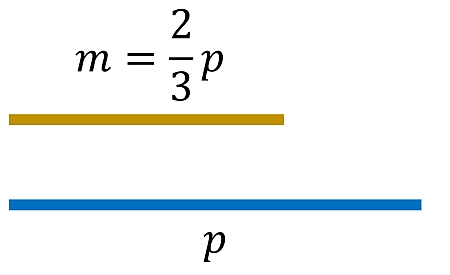# Mike has two-third of the minutes that Payton has. Create a diagram illustrating the...

## Question:

Mike has two-third of the minutes that Payton has. Create a diagram illustrating the relationship. Payton has {eq}\rule{1cm}{0.15mm} {/eq} times as many minutes as Mike.

## Algebraic Equations:

An Algebraic Equation is the first step to the language of Mathematics. It involves the use of variables represented with lowercase alphabets usually. It helps to represent or formulate the problem in hand using algebra and then solve it using simple arithmetic operations.

The first step to formulate any problem in mathematics will be to define necessary variables. Let's say Mike has {eq}m {/eq} minutes and Payton has {eq}p {/eq} minutes. As we denote them.

This can be represented diagrammatically as follows. Consider the minutes on a time scale. So, Mike has {eq}m {/eq} minutes as represented by the yellow line while Payton's minutes are represented by the blue line.Now it is mentioned in the question that Mike has {eq}\dfrac{2}{3}^{rd} {/eq} of the minutes that Payton has.

So, this becomes, {eq}m=\dfrac{2}{3}~p {/eq}.

The question is to find the minutes that Payton has as compared to Mike.

Multiplying both the sides by {eq}\dfrac{3}{2} {/eq} to make {eq}p {/eq} as the subject.

We get, {eq}p=\dfrac{3}{2}~m=1.5\times{m} {/eq}.

So the final answer line becomes as follows...

Payton has {eq}\underline{1.5} {/eq} times as many minutes as mike.